# 17 Electric Charge Electric Filed Nature of Electric

• Slides: 30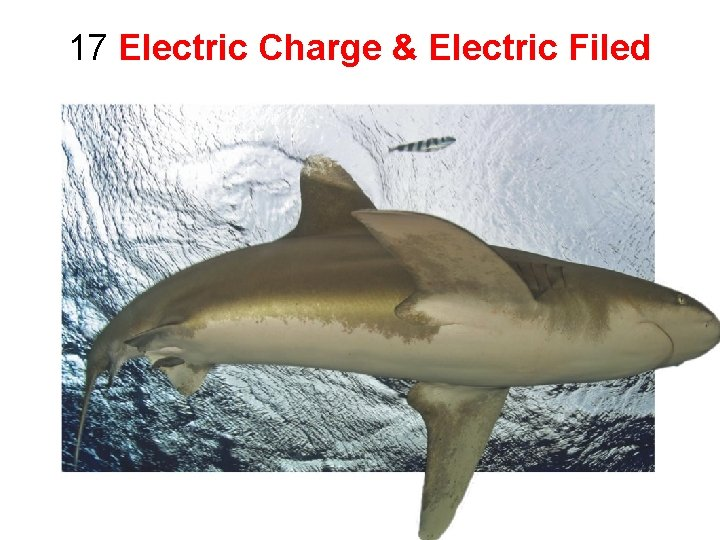17 Electric Charge & Electric Filed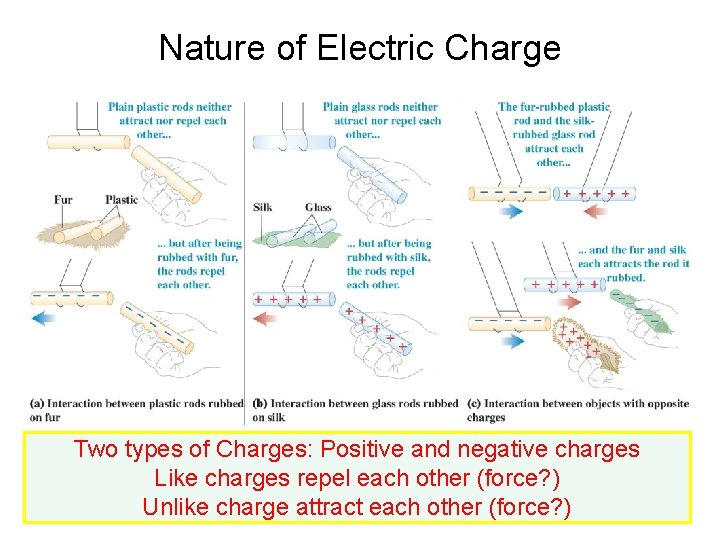Nature of Electric Charge Two types of Charges: Positive and negative charges Like charges repel each other (force? ) Unlike charge attract each other (force? )Physical Basis of Electric Charge All the atoms are electrically neutral (ions, ionization & oxidization) Same number of protons & electrons Different number of neutrons: IsotopesConductors & Insulators If charges can move freely in a materials—conductor If charges can’t move in a materials—insulator Semiconductor, Limits, and Heat transfer Metals are good conductors What about water?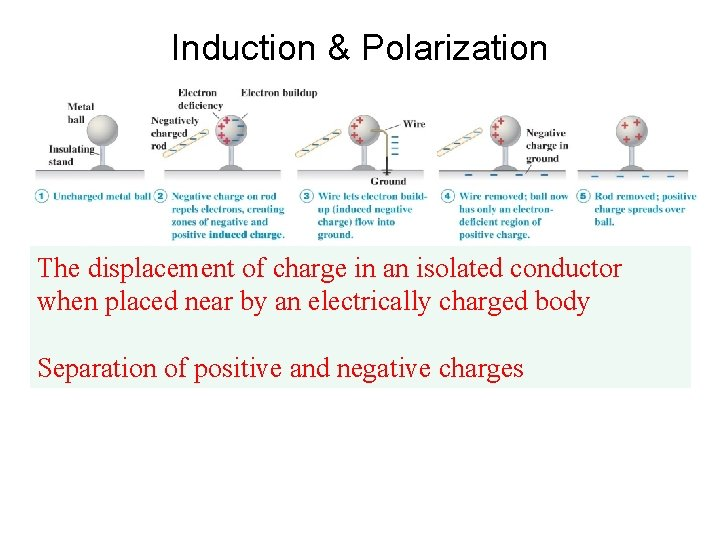Induction & Polarization The displacement of charge in an isolated conductor when placed near by an electrically charged body Separation of positive and negative charges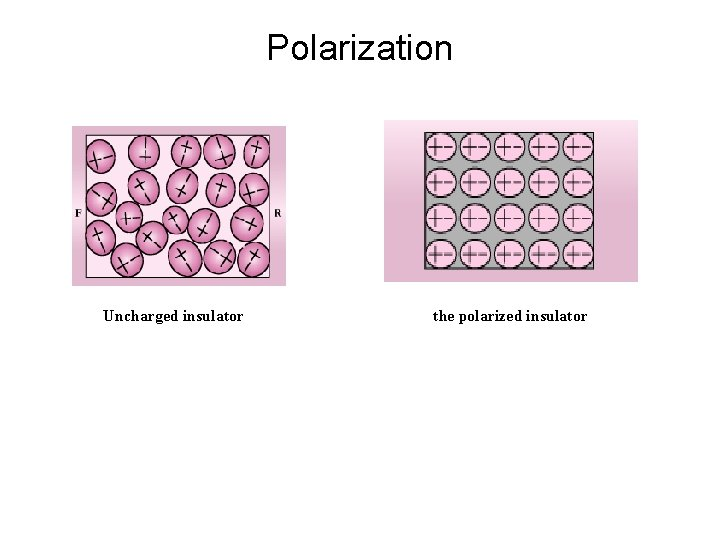Polarization Uncharged insulator the polarized insulatorConservation & Quantization of Charge Conservation: Total electric charges in any closed system is a constant. Charge can be transferred from on object to another. Quantization: minimum amount of charge: e, the basic unit, the charge of the electron or proton. Any Q = integer x e e = 1. 602 x 10 -19 C or 1 C ~ 6 x 1018 protons(+) or electrons(-)! m. C, n. C…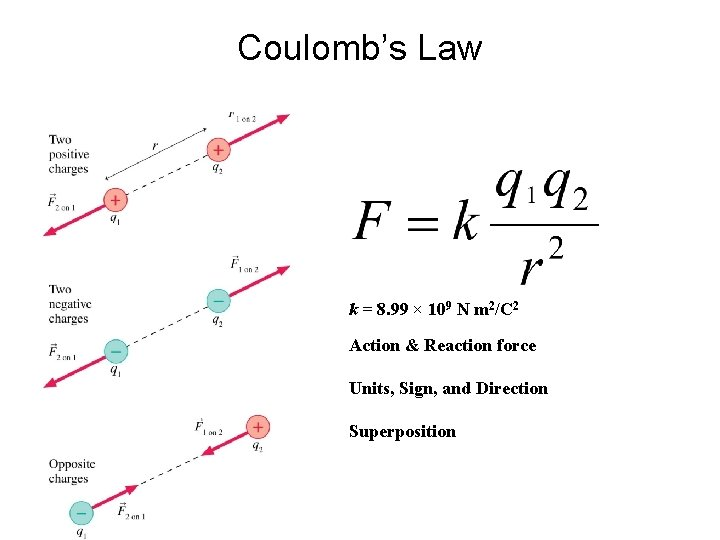Coulomb’s Law k = 8. 99 × 109 N m 2/C 2 Action & Reaction force Units, Sign, and Direction SuperpositionElectric Field & Electric Forces Definition of Electric Field: Unit: N/C Direction ?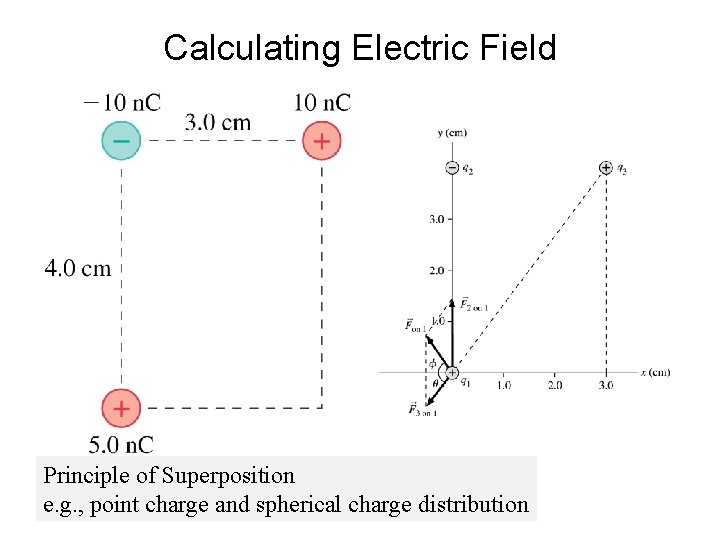Calculating Electric Field Principle of Superposition e. g. , point charge and spherical charge distributionElectric Field Lines— Physical Meaning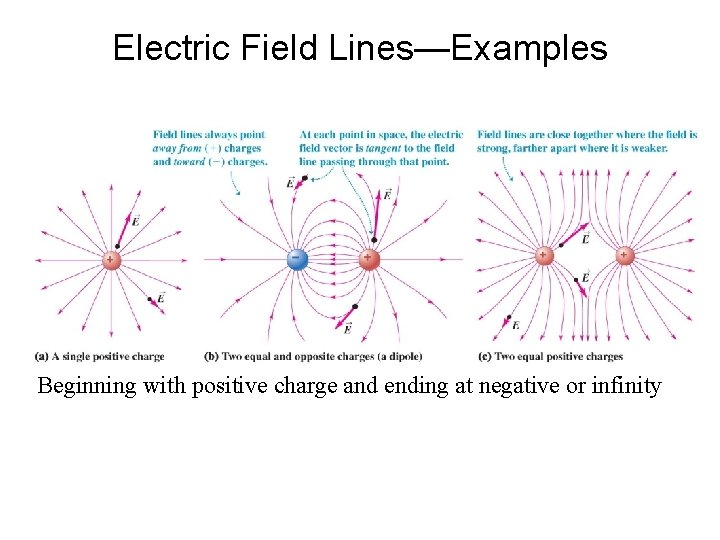Electric Field Lines—Examples Beginning with positive charge and ending at negative or infinityElectric Field Lines—Examples + + + -*Gauss’s Law & Field Calculation Electric Flux: Example: point charge*Charges on ConductorsSummary: Charge, Conductors & Insulators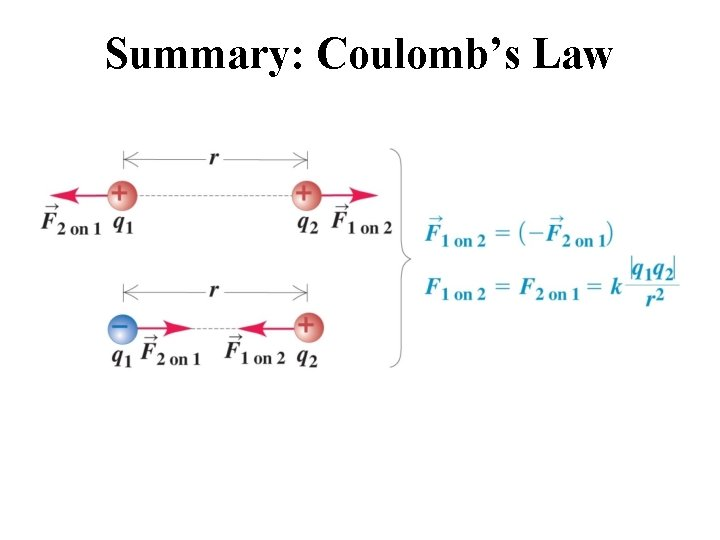Summary: Coulomb’s Law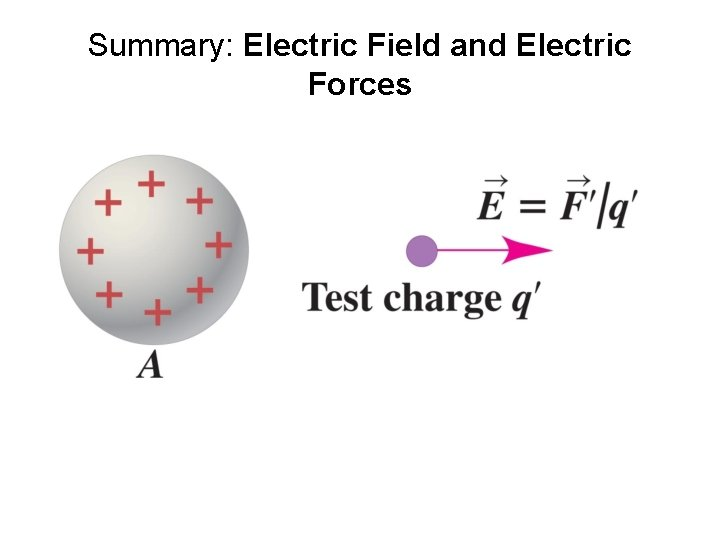Summary: Electric Field and Electric Forces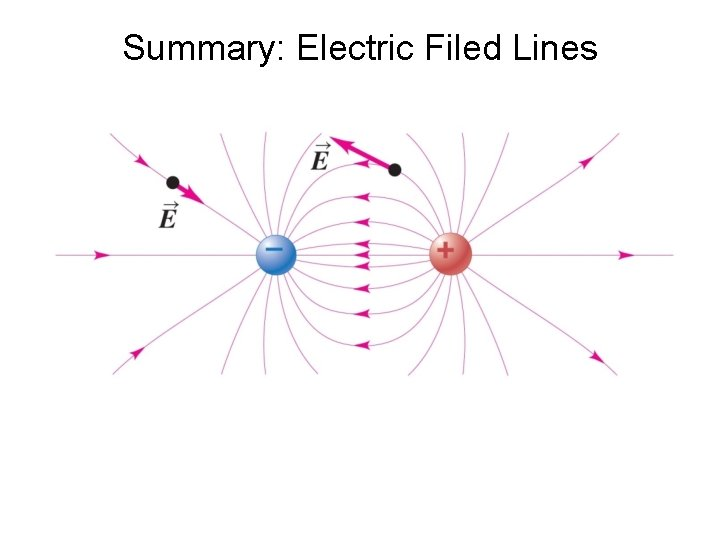Summary: Electric Filed LinesSummary: Gauss’s Law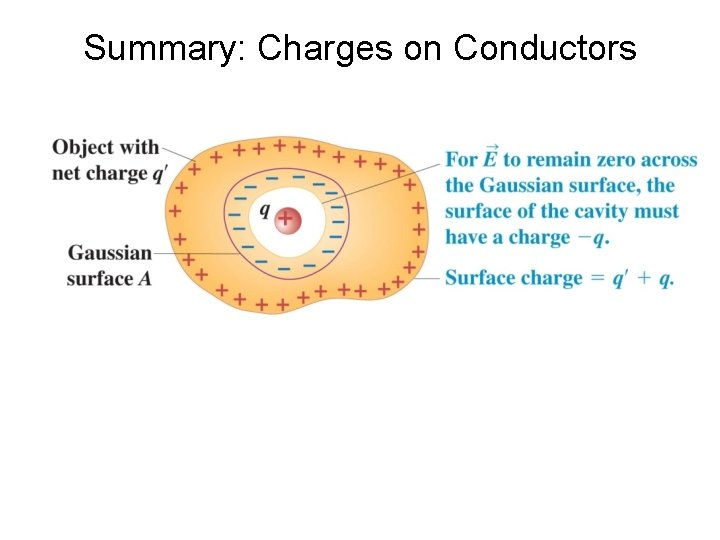Summary: Charges on Conductors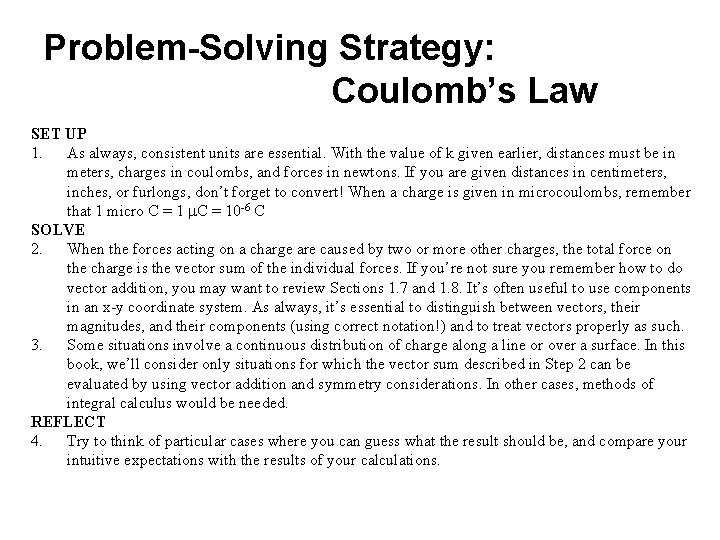Problem-Solving Strategy: Coulomb’s Law SET UP 1. As always, consistent units are essential. With the value of k given earlier, distances must be in meters, charges in coulombs, and forces in newtons. If you are given distances in centimeters, inches, or furlongs, don’t forget to convert! When a charge is given in microcoulombs, remember that 1 micro C = 1 m. C = 10 -6 C SOLVE 2. When the forces acting on a charge are caused by two or more other charges, the total force on the charge is the vector sum of the individual forces. If you’re not sure you remember how to do vector addition, you may want to review Sections 1. 7 and 1. 8. It’s often useful to use components in an x-y coordinate system. As always, it’s essential to distinguish between vectors, their magnitudes, and their components (using correct notation!) and to treat vectors properly as such. 3. Some situations involve a continuous distribution of charge along a line or over a surface. In this book, we’ll consider only situations for which the vector sum described in Step 2 can be evaluated by using vector addition and symmetry considerations. In other cases, methods of integral calculus would be needed. REFLECT 4. Try to think of particular cases where you can guess what the result should be, and compare your intuitive expectations with the results of your calculations.Problem-Solving Strategy: Electric Field Calculations SET UP 1. Be sure to use a consistent set of units. Distances must be in meters, charges in coulombs. If you are given cm or n. C, don’t forget to convert. 2. Usually, you will use components to compute vector sums. As we suggested for problems involving Coulomb’s law, it may be helpful to review Sections 1. 7 and 1. 8. Use proper vector notation; distinguish carefully between scalars, vectors, and components of vectors. Indicate your coordinate axes clearly on your diagram, and be certain that the components are consistent with your choice of axes. SOLVE 3. In working out directions of vectors, be careful to distinguish between the source point S and the field point P. The field produced by a positive point charge always points in the. direction from source point to field point; the opposite is true for a negative point charge. REFLECT 4. If your result is a symbolic expression, check to see whether it depends on the variables in the way you expect. If it is numeric, estimate what you expect the result to be and check for consistency with the result of your calculations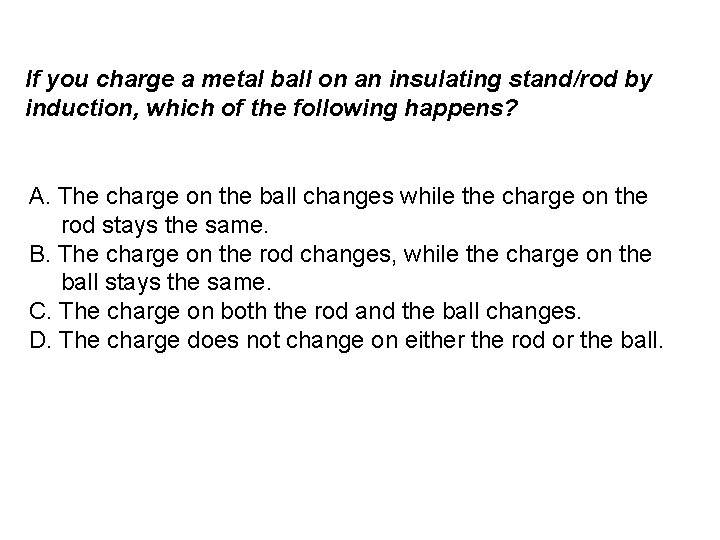If you charge a metal ball on an insulating stand/rod by induction, which of the following happens? A. The charge on the ball changes while the charge on the rod stays the same. B. The charge on the rod changes, while the charge on the ball stays the same. C. The charge on both the rod and the ball changes. D. The charge does not change on either the rod or the ball.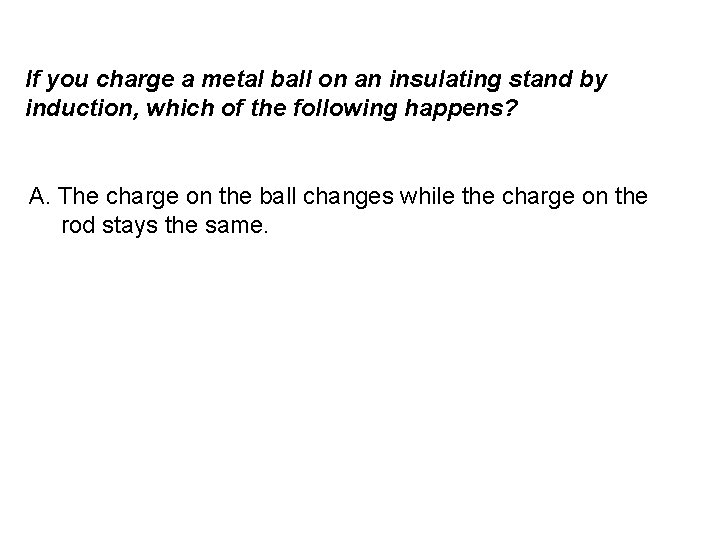If you charge a metal ball on an insulating stand by induction, which of the following happens? A. The charge on the ball changes while the charge on the rod stays the same.A small object with a charge of magnitude q creates an electric field. At a point 1. 0 m to the north of the charge, the field has a value of 2. 0 N/C directed south. At a point 0. 5 m to the west of the charge the field has a value of: A. 4. 0 N/C directed east B. 4. 0 N/C directed west C. 8. 0 N/C directed east D. 8. 0 N/C directed west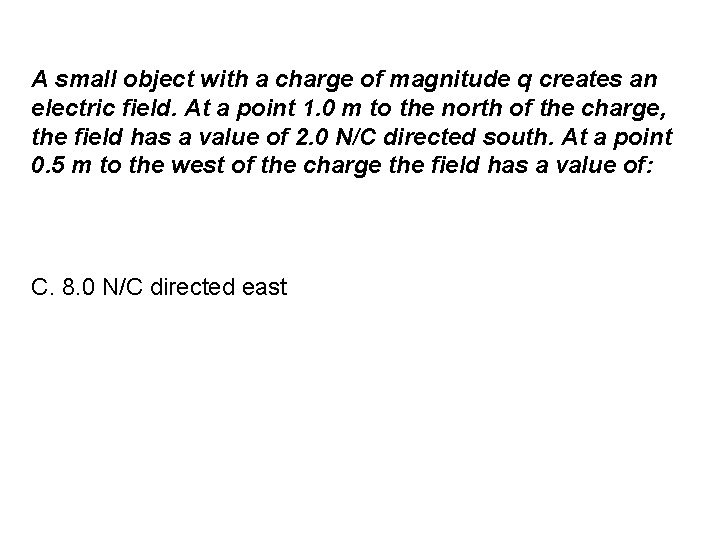A small object with a charge of magnitude q creates an electric field. At a point 1. 0 m to the north of the charge, the field has a value of 2. 0 N/C directed south. At a point 0. 5 m to the west of the charge the field has a value of: C. 8. 0 N/C directed eastExample 17. 2: Gravity & Electric ForceProblem-SolvingHomework Ch 17: 1, 3, 5, 7, 14, 20, 31, 34, 40, 50.